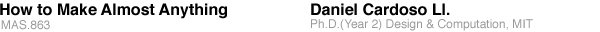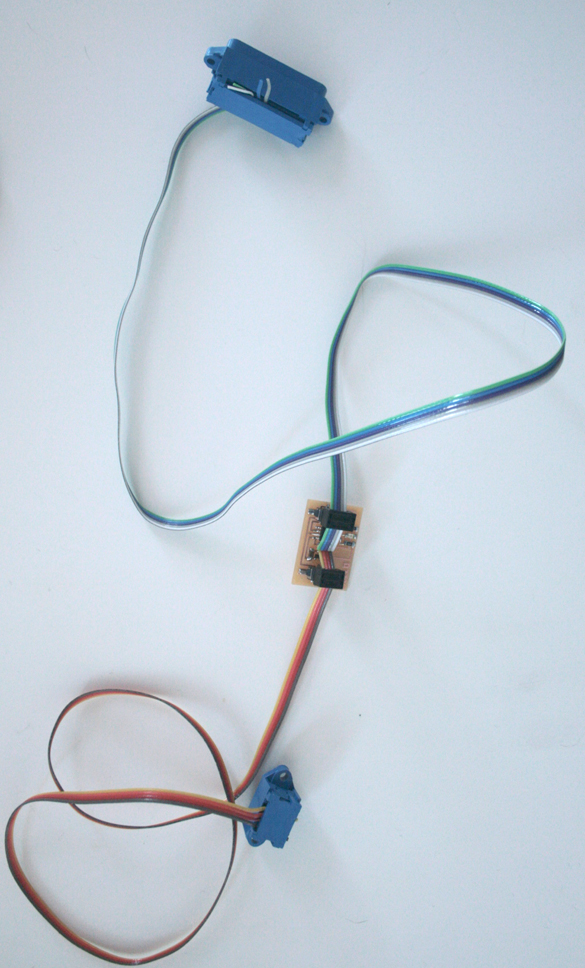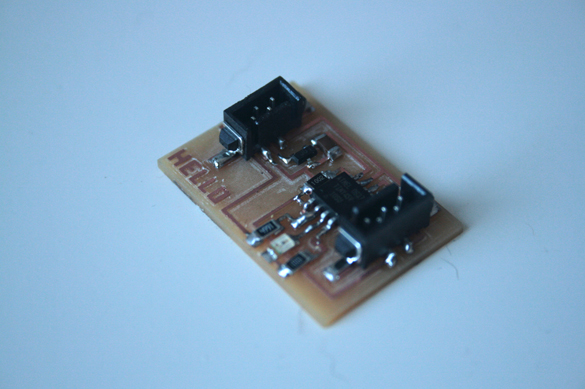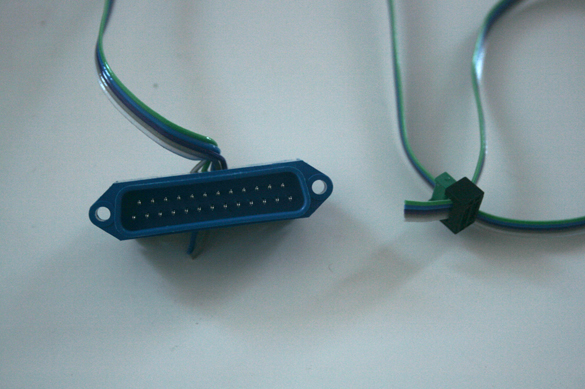sections contact email: dcardoso [at] sinmit [dot] edu

## MicroController Programming

The idea for tis interactive object is to have a player guess a number randomly chosen by the computer. The LED will blink faster when the number entered by the player is closer to the random one. Because of the way I structured the code I ran into control-flow problems (I think need a thread that 'listens' to the keyboard, and a different one that keeps the LED blinking at varying speeds.

I wrote the code in C, and generated the HEX file using WinAVR. For loading the code I followed the following sequence of steps:

1. Connect the board to the serial (power) and parallel (programming) ports in the lab's computer. See below for more information on the cables.

2. From Shell, give power to the circuit

python rx.py /dev/ttyS0 9600

3. Burn program iinto the microcontroller

avrdude -p t45 -c bsd -U flash:w:myprogram.hex

4. Disconnect parallel port

6. Open a python terminal

python term.py /dev/ttyS0 9600The C Program
[buggy]

int main(void) {

unsigned char rxbyte;
output(tx_pin);
output(led_pin);
clear(tx_pin);

char startgame[] PROGMEM = "Find the random number";
char winmessage[] PROGMEM = "You have won!";

print_string(startgame);
put_char('/n');

int r = 8; //my_random();
//print_string(r);
int interval = 2000;

int counter = 0;
int v;
int n;

while (1){

int vTemp = v;
set(led_pin);
_delay_ms(10);
clear(led_pin);
_delay_ms(interval);

}

rxbyte = get_char(); // this prevents the program to flow
v = rxbyte;
n = (abs)(rxbyte - r);

if (range == 1){
interval = 500;
}

if (range == 2){
interval = 200;
}

if (range == 3){
interval = 50;
}

}

I realized that for implementing this I would need to implement different threads. I plan to fo this during this week.Gradient

What did work was a gradient 'routine' for the LED.

void blink_gradient(){
//
// blinks the LED at an increasing speed
//

int t = 0; // steps
int interval = 2000;

while (t < 100){

blink();

_delay_ms(interval);

if ( t < 30 ) {
interval = interval - 200;
}

if ((t > 30)&&(t < 60)){
interval = interval - 100;
}

if ((t > 60) && (t < 90)){
interval = interval - 25;
}

if ( t > 90 ){
interval = interval - 10;
}

t = t + 1;

}

char m[] PROGMEM = "End of gradient.";

set(led_pin);
_delay_ms(2000);
clear(led_pin);
//print_string(m);
put_char('\n');

}[ daniel cardoso site ]